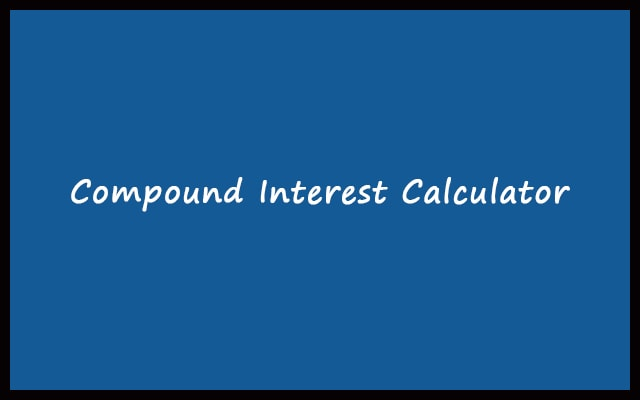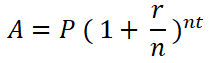# Compound Interest Calculator with Formula (daily, weekly, monthly, yearly)

Compound interest is a common term in the financial world. It is the total amount to pay. In other words, it’s an addition of principal amount and interest. Use this Compound Interest Calculator to find it.

\$

%

\$

\$

\$

\$

%

Period No. Payment Principal Sum
RelatedYou can easily estimate the total amount after some years using the Compound Interest Formula. The Compound Interest formula also gives you the relationship between different factors like time.

Our tool uses the same formula to estimate the total amount after the given duration of the period. Furthermore, it is very easy to use. Even more, the article has all the information regarding the tool. How to use it? What are the advantages? All about the Compound Interest Calculator.

## Compound Interest Formula

The formula to calculate Compound Interest:Where,
A = Final value/amount
P = Initial unpaid balance
r = Interest value/rate
n = Number of times the interest value applied per time period
t = Time period in which interest rate applied

First of all, Let’s look at some examples. To better understand how’s compound interest is calculated. Then we will move on to some applications.

##### Example 1:

Given that the Principal amount is 1500. The bank gives an annual interest of 4.3%. The interest is compounded quarterly. You need to find the total amount after 6 years.

A = P(1 + r/n)nt

We have,

P = 1500
r = 0.043
n = 4
t = 6

Putting these values in the formula we get,

A = 1500(1 + 0.043/4)4 x 6

A = 1938.8368

The total amount after 6 years will be approximately 1938.8

To determine the interest amount: Find the difference between the compounded amount and the principal amount. In this case, Interest amount = 1938 – 1500 = 438. This is the interest received.

##### Example 2:

Given that the Principal amount is 2000. The bank gives an annual interest of 6.3%. The interest is compounded quarterly. You need to find the total amount after 4 years.

Similarly, Using the same Compound Interest formula, we will solve the above problem.

A = P(1 + r/n)nt

We have,

P = 2000
r = 0.063
n = 4
t = 4

Putting these values in the formula we get,

A = 2000(1 + 0.063/4)4 x 4

A = 2568.143

The total amount after 4 years will be approximately 2568.

Consequently, you can find the interest amount. Just find the difference between the compound amount and the principal amount. It will be 2568.143 – 2000 = 568.143.

## Uses of Compound Interest Calculator

• Compound Interest is useful in generating profits. Also, It opens doors for business profits. In addition, investors can estimate the outcome. In this way, they increase the company’s profit. Hence, the compound interest calculator is a business tool and useful to increase profits exponentially.
• During an investment, It is very important to know whether the investment will yield profit or loss. Taking calculated risk is advised. Hence, the compound interest calculator is in use to ensure benefit or loss. Now, you are ready to invest.
• Calculation Pension payments also sometimes may require CI calculation. Pension is an amount given to an employee. When he or she retires from a company. It is part of the money collected from his or her salary. Whenever someone gets his or her pension. There is an option to invest the amount. In order to estimate the compound interest. Use the best Compound Interest Calculator to manage all the things safely.